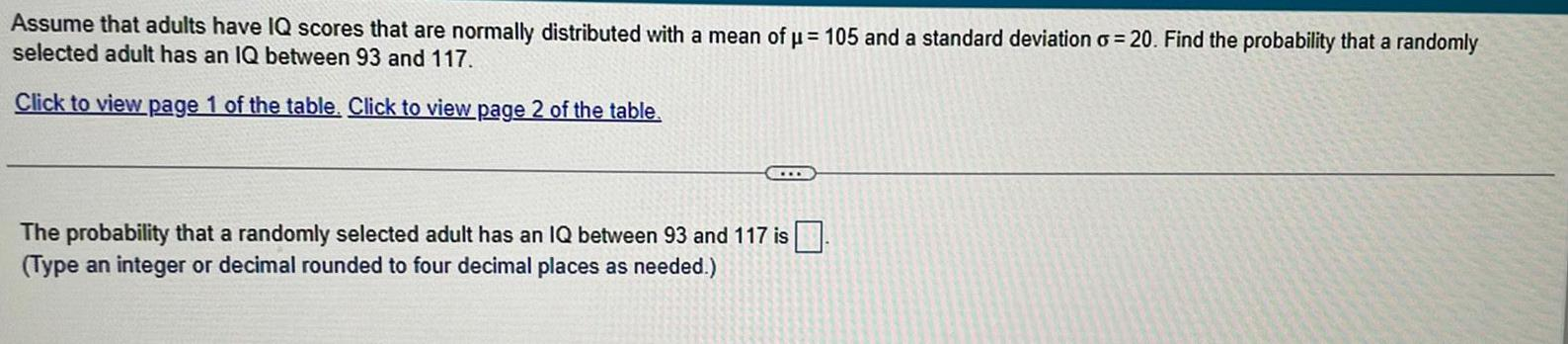Question:

# Assume that adults have IQ scores that are normally

Last updated: 7/7/2022Assume that adults have IQ scores that are normally distributed with a mean of μ = 105 and a standard deviation o=20. Find the probability that a randomly selected adult has an IQ between 93 and 117. The probability that a randomly selected adult has an IQ between 93 and 117 is (Type an integer or decimal rounded to four decimal places as needed.)